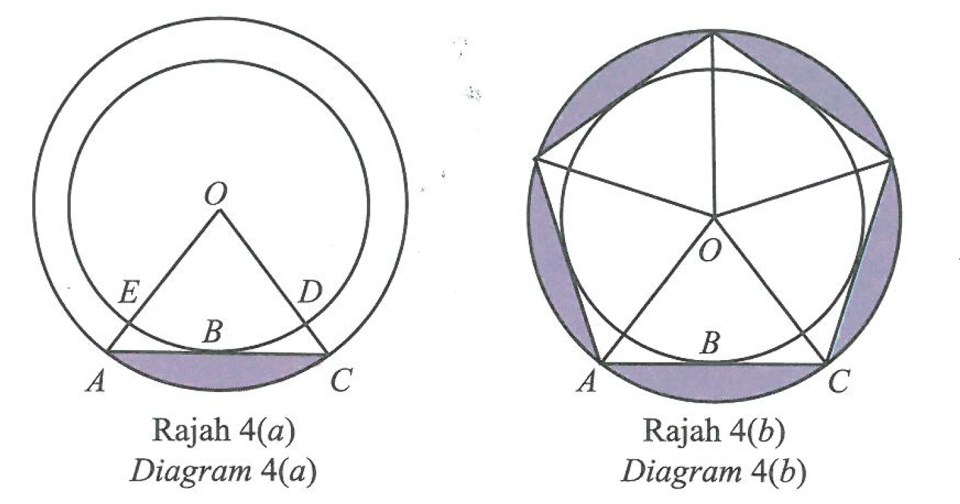\

# SPM 2021 Add Maths Trial Paper (Selangor) – Paper 2 (Question 5)

Question 5:
Diagram 4(a) shows two concentric circles with the centre O. ABC is a tangent to the smaller circle at B. Five triangles OAC form a regular pentagon inscribed the bigger circle as in Diagram 4(b).By using π = 3.142,
(a) show that the length of OA = 9.889 cm, given that OD = 8 cm. [1 mark]

(b) calculate
(i) the difference between the length, in cm, of arc AC and arc⁡ DE.
(ii) the area, in cm2, of the blue coloured region in Diagram 4(b).
[6 marks]

Solution:
(a)

(b)(i)

(b)(ii)